##### Statistics: 1001 Practice Problems For Dummies (+ Free Online Practice)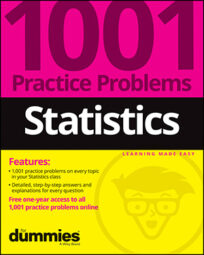For these three sample questions, consider that: A researcher conducted an Internet survey of 300 students at a particular college to estimate the average amount of money students spend on groceries per week. The researcher knows that the population standard deviation of weekly spending is \$25. The mean of the sample is \$85.

The following table provides the z*- values for selected (percentage) confidence levels.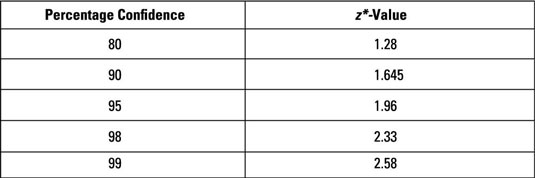## Sample questions

1. What is the margin of error if the researcher wants to be 99% confident of the result?

The formula for margin of error when estimating a population mean is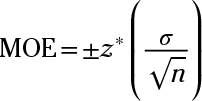where z* is the value from the table for a given confidence level (99% in this case, or 2.58),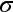is the standard deviation (\$25), and n is the sample size (300).

Now, substitute the values into the formula and solve: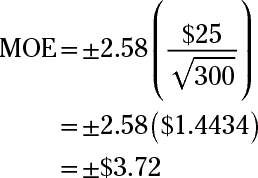The margin of error for a 99% confidence interval for the population mean is plus/minus \$3.72.

2. What is the margin of error if the researcher wants to be 95% confident in the result?

The formula for margin of error when estimating a population mean iswhere z* is the value from the table for a given confidence level (95% in this case, or 1.96),is the standard deviation (\$25), and n is the sample size (300).

Now, substitute the values into the formula and solve: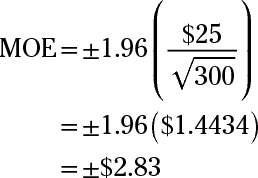The margin of error for a 95% confidence interval for the population mean is plus/minus \$2.83.

3. What is the lower limit of an 80% confidence interval for the population mean, based on this data?

To find the lower limit for the 80% confidence interval, you first have to find the margin of error. The formula for the margin of error when estimating a population mean iswhere z* is the value from the table for a given confidence level (80% in this case, or 1.28),is the standard deviation (\$25), and n is the sample size (300).

Now, substitute the values into the formula and solve: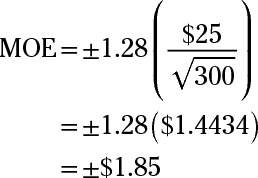Next, subtract the MOE from the sample mean to find the lower limit: \$85.00 – \$1.85 = \$83.15.

If you need more practice on this and other topics from your statistics course, visit 1,001 Statistics Practice Problems For Dummies to purchase online access to 1,001 statistics practice problems! We can help you track your performance, see where you need to study, and create customized problem sets to master your stats skills.